The Vietnamese Mathematical Hobby Group

MATHEMATICAL TEST PAPER #18

Question 1:

(i) Show that the curves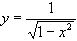and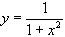each have a stationary point at the point A(0, 1). Hence, sketch these curves on the same diagram.

(a) Determine the area bounded by the two curves, and the ordinates x = +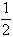.

(b) The straight line y = 1/2 cuts the curveat B, C. The arc BAC of this curve is rotated about the y-axis. Find the volume of the solid so generated.

(ii) Assume that for all real numbers x and all positive interger n,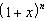=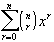Show that (a)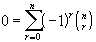and find the expression for (b)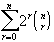(c)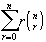.

Question 2:

(i) If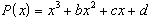is an odd function (i.e.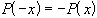), show that b = d = 0. Hence, prove that the curve y = P(x) always passes through the origin.

(ii) Find a monic polynomial Q(x) of degree 3 so that Q(x) is an odd function and has a root at x = -2. State whether there is any other polynomials of degree 3 with these properties of is Q(x) unique.

(iii) A spherical bubble is expanding so that its volume is increasing at the constant rate of 10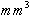per second. What is the rate of increase of the radius when the surface area is 500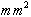(note that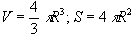). (1984/Q4)

Question 3:

(i) Using the definition of derivative on the function y =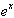, show that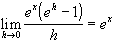. And, hence obtain the result for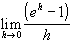.

(ii) Use the appropriate series formula to find an expression for the sum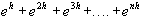, where nh = 1.

(iii) It is given that A>0, B>0 and n is a positive integer.

(a) Divide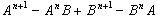by A - B and deduce that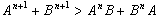.

(b) Use (a) and mathematical induction to prove that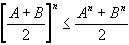.

Question 4:

Particles P and Q are thrown at the same instant from a point O in a horizontal plane, each with the same velocity V. The particle P is throwsn vertically and the particle Q is projected elevation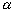. Using calculus prove that the difference in the

(a) greatest heights attained above the horizontak plane is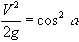;

(b) times taken to return to the horizontal plane is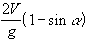;

(c) ranges on the horizontal plane is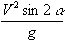.

If Q is projected at such an angle that the differene in the horizontal range is three times the difference in the greatest heights, find the angle of projection.

If with this angle, the difference in horizontal range is 240 m, find the exact speed of projection of P and Q. Take g = -10 m/s2.

Question 5:

(i) Find the equation of the chord of contact of the tangents from the point T(3, -2) to the parabola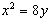, and show that this chord of contact passes through the focus of the parabola.

(ii) Use the factor theorem to find a factor of the polynomial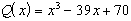, hence express Q(x) as the product of 3 linear polynomials.

(iii) Find the equation of the curve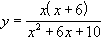in the new (X, Y) coordinate system with origin at x = -3, y = 1 and the axes are unchanged in direction.

(iv) A family of curves have slope given by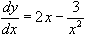. Find the equation of the equation of that member of the family which passes through the point (1, -2).

Question 6:

(i) Find the coefficient of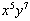in the expansion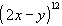.

(ii) Find the second derivative of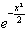; hence, determine the value of x the curve y =is concave upwards.

(iii) A box contain13 counters numbered 1 to 13.

(a) Two counters are drawn at random, the first being replaced before the second counter is drawn. What is the joint probability that the first counter bears an even number and the second counter bears an odd number ?

(b) What is the probability if the first counter is NOT replaced before the second drawing is made ?

Question 7:

(i) Find a primitive function of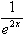. Hence, determine the area under the curve y =for 1 < x < 1.5.

(ii) Sketch the graph of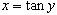, and on a separate diagram, show clearly the graph of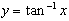. What is the domain and the range of the function.

(iii) Find the derivative ofand hence obtain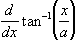. What is the relationship between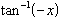and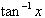.

(iv) Use the result of (iii) to find the exact value of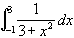.

(v) If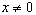, prove that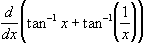= 0, and hence deduce the value of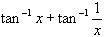.